Question

At the beginning of summer, Mark has $200 in savings and Ryan has$350. The brothers have mowing jobs set up for the summer. Because Mark is older, he receives $20 for each mowing job and Ryan receives$15. The brothers’ parents pay for the fuel used to mow, so all of the money the brothers earn goes to savings. Write two equations, one for Mark and one for Ryan, to represent the money in savings (s) based on the number of mowing jobs (j) Mark and Ryan do. Determine the number of mowing jobs it takes for Mark to have the same amount in savings as Ryan does. Show your work.

1.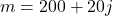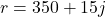Number of jobs is 30

Step-by-step explanation:

Variable representation

Let Mark’s total earnings for moving be m

Let Ryan’s total earnings for moving be r

Represent the number of jobs with j

Setup equation

Mark: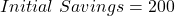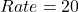So, the total savings is:Ryan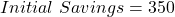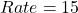So, the total savings is:To get the number of jobs they save the same amount.

We have: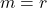Substitute values for m and r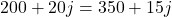Collect Like Terms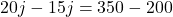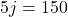Divide both sides by 5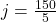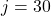Hence, the number of jobs is 30